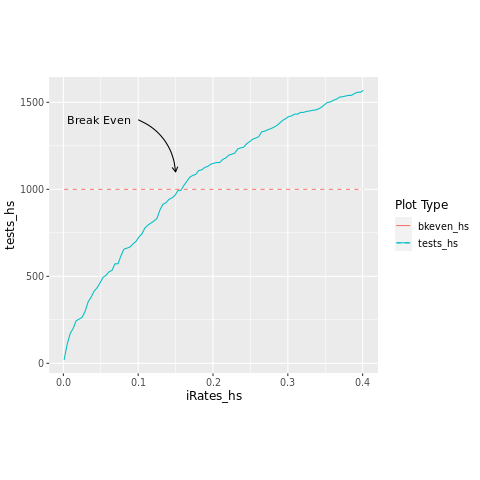In :
:ext QuasiQuotes
import qualified H.Prelude as H
H.initialize H.defaultConfig
[r| require("ggplot2") |]

Loading required package: ggplot2
0x00007f53c400a1c0

## Herman Idea¶

Herman called me and asked if I could determine if his Covid19 testing idea was feasible.

The idea was the following:

• Take a set of patients
• Take their blood
• Divide the set into two halves
• Mix a portion of blood from each patient in each half together
• Test each half
• If a subsample (half) tests negative discard and notify appropriate patients they are negative
• If a subsample (half) tests positive divide it in half again and, using another portion of their blood, mix the blood in each half, and test again. -- Do this recursively until every half tested negative or contained only one patient.

Again we are trying to minimize the number of tests.

Let $N$ be the number of cool patients
Let $R$ be the infection rate

Testing everyone requires,

$$T_{e} = N\ \ \ tests$$

#### Herman Techinque¶

The Herman technique requires knowledge of the infection rate to determine the number of tests required.

The maximum number of tests required is

\begin{equation} T_{H}\ =\ \sum_{k=1}^{log_2(N)} 2^k \end{equation}
In [ ]:



But in general the number of tests required would be,

\begin{equation} T_{H}\ =\ \ f(N,R) \end{equation}

where $f$ is a probabilistic representation of the distribution of test outcomes

##### Example¶

Let $N = 1024$

Traditional testing would require 1024 tests every time. The Herman technique would require 2046 tests maximum and 2 tests at a minimum. It's effectiveness is determined by $R$, the infection rate.

$T_H=2\ \ \ \ \ for\ \ R=0$

and

$T_H=2046\ \ \ \ \ for\ \ R=1$

##### Determine Break Even Point¶

To determine the break even point between traditional testing and the Herman technique a Haskell program was written. A plot will be generated for $R$ vs $N$.

In :
import System.Random
import qualified Data.List.Split as DL

genRands :: Double -> Double  -> [Double]
genRands low high = randomRs (low, high) (mkStdGen 41)

asBools :: Double -> [Double] -> [Bool]
asBools iRate = map (<= iRate)

bSplit :: [Bool] -> ([Bool], [Bool])
bSplit myList = splitAt ((length myList + 1) div 2) myList

hermanPlotData :: Int -> [Double] -> [(Double,Double)]
hermanPlotData count  =
map (\r -> (fromIntegral (hermansMethod (bRands r count))::Double, r))

hermansMethod :: [Bool] -> Int
hermansMethod bs =
let
(left,right)  = bSplit bs
hitsLeft  = sum $map fromEnum left hitsRight = sum$ map fromEnum right
in
whatToDo left hitsLeft + whatToDo right hitsRight

where

whatToDo :: [Bool] -> Int -> Int
whatToDo samples hits
| hits == 0 = 1
| length samples <= 1 = 1
| otherwise = 1 + hermansMethod samples

bRands :: Double -> Int -> [Bool]
bRands iRate patientCount = take patientCount (asBools iRate (genRands 0.0 1.0))


This is an example of the initial blood distibution. The splitting of tests is always done left to right

In :
hermansMethod [False,True,True,False,True,False,False,True]

14
In :
hermansMethod [False,False,False,False,True,True,True,True]

8

Note the same number of positive results, just the order has changed.

Now let's setup the simulation and plot.

In :
let patients = 1000 -- Patient count (limited to 10^6)
let infectionRates = [0.001,0.005..0.4]
let bkevens = take (length infectionRates) (repeat 1000.0)
let (tests,iRates) = unzip \$ hermanPlotData patients infectionRates


coord_fixed(ratio=(1.5)) +

In :
[rgraph|
d <- data.frame(iRates_hs,tests_hs,bkevens_hs)
ggplot(d, aes(iRates_hs)) +
theme(text=element_text(family="Ariel", size=12), aspect.ratio=0.9) +
geom_line(aes(y = tests_hs, color = "tests_hs")) +
geom_line(aes(y = bkevens_hs, color = "bkeven_hs"), linetype="dashed") +
labs(color = "Plot Type") +
annotate( geom = "curve", x = 0.1, y = 1400, xend = 0.15, yend = 1100,
curvature = -.3, arrow = arrow(length = unit(2, "mm"))) +
annotate(geom = "text", x = 0.09, y = 1400, family = "ariel", label = "Break Even", hjust = "right") |]